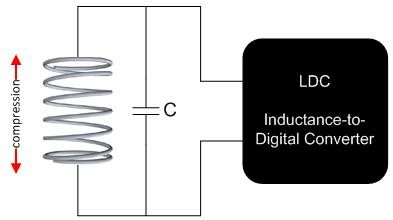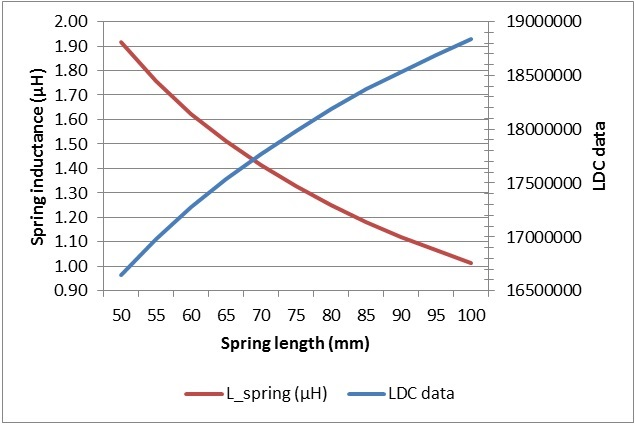# Inductive sensing: How to sense spring compression

While most inductive-sensing applications use either printed circuit board (PCB) coils or wire-wound inductors as the sensor, inductance-to-digital converters (LDCs) can use almost any inductor as a sensor – even a spring. Springs are useful as sensors because the spring’s inductance varies directly with changes in length or other physical changes. Figure 1 shows how to connect a spring to an LDC.Figure 1: Spring used as a sensor by an LDC

To evaluate using a spring as a sensor, I used the LDC1612EVM evaluation module to measure the inductance of a spring as I extended the spring across a range of lengths. To do this, I first removed the on-board sensor from the EVM and replaced it with a spring. The spring was made of 0.7mm-thick steel, had 46 turns and a diameter of 7.3mm. Figure 2 shows the spring that I connected to the EVM.

Figure 2: Spring setup

The inductance of my spring is too low to be used as a sensor for the LDC1612 on its own, so I added a 2.2μH fixed wire-wound surface-mount device (SMD) inductor in series. (For details on how to use a series inductor to increase sensor impedance, see my blog post “How to use a tiny 2mm PCB inductor as a sensor.”) With a 1nF sensor capacitor, the oscillation frequency was 2.5MHz. Figure 3 shows the sensor components that I used.

Figure 3: Sensor components

I stretched the spring from 50mm to 100mm in 5mm increments and measured LDC1612 output data at each step. From the data, I calculated the sensor inductance using Equation 1:

where

and fref = reference clock (40MHz on the LDC1612 EVM).

Figure 4 shows the data and spring inductance after subtracting the 2.2μH series inductor.Figure 4: LDC1612 data and spring inductance versus spring length

The data samples that I collected when extending the spring from 50mm to 100mm in 5mm steps are monotonic and can be used to precisely determine the length of the spring. During this spring-compression range, the inductance decreases from 1.92μH (LDC output 16,644,000) to 1.01μH (LDC output 18,840,000). Thus, over this range, stretching the spring by 1μm results in a 44-codes increment in the LDC1612 data output on average.

This data shows that you can use inductive sensing to directly measure the inductance shift that results from compressing a spring, and that springs can serve as an alternative sensor to PCB coils and wire-wound inductors.